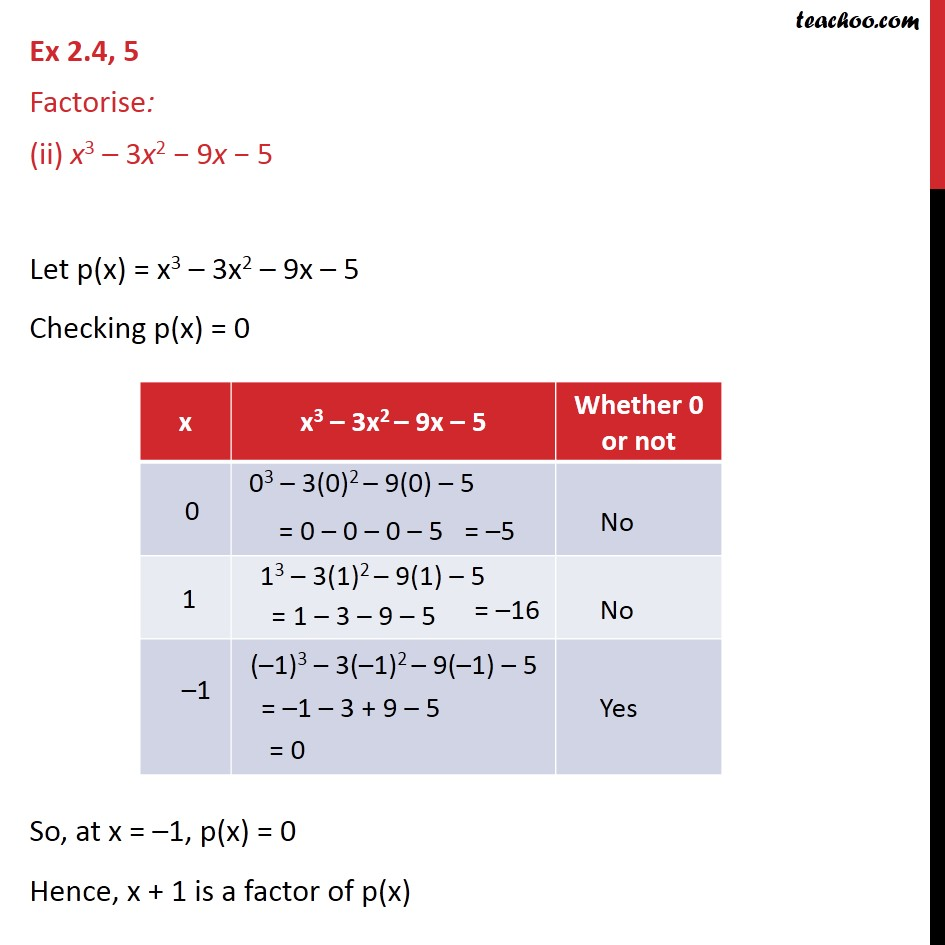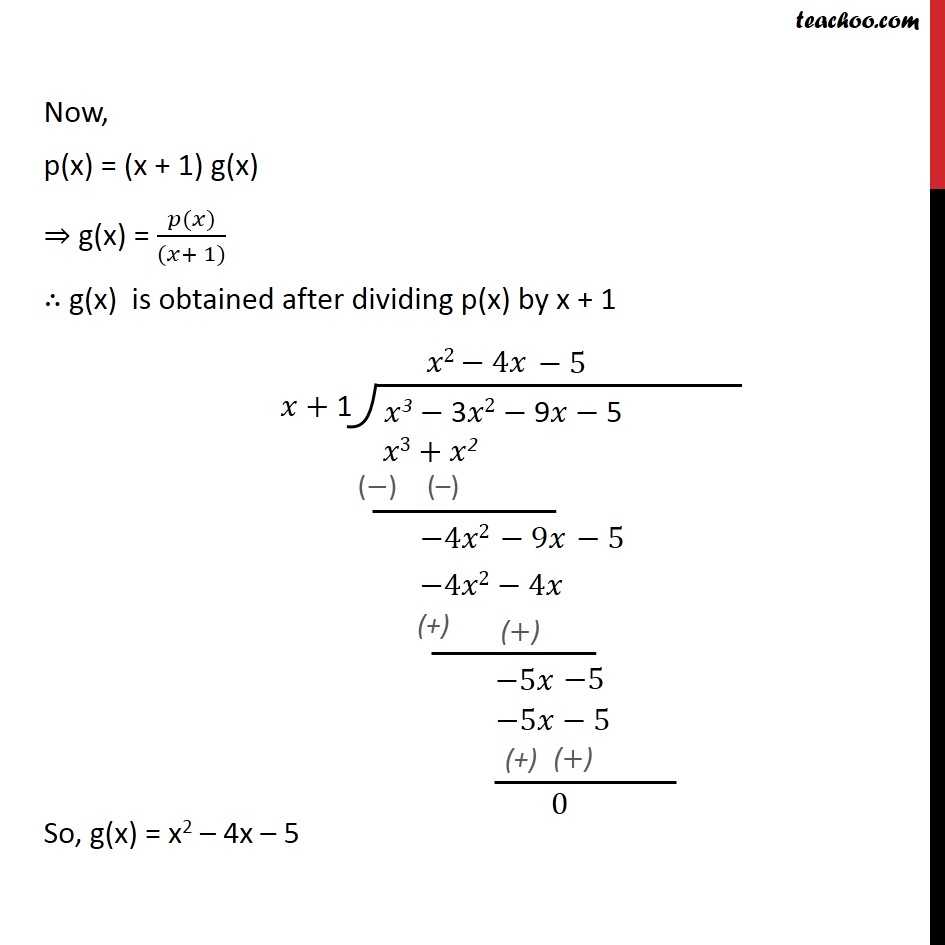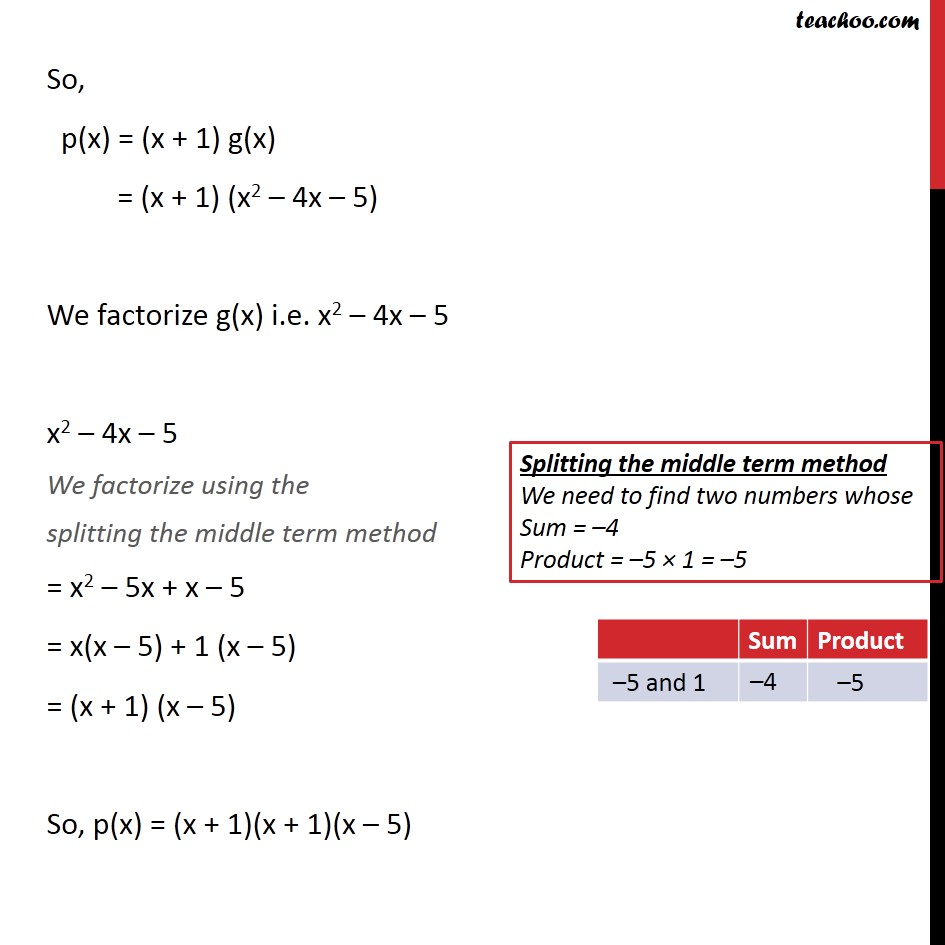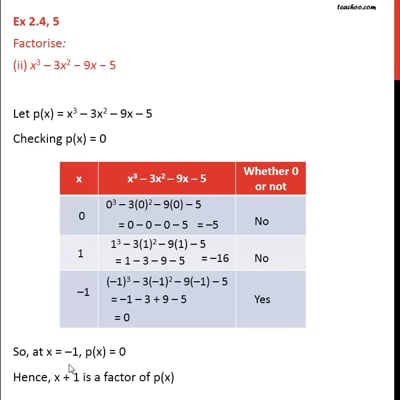Ex 2.4

Chapter 2 Class 9 Polynomials
Serial order wiseThis video is only available for Teachoo black users

Maths Crash Course - Live lectures + all videos + Real time Doubt solving!

### Transcript

Ex 2.4, 5 Factorise: (ii) x3 – 3x2 − 9x − 5 Let p(x) = x3 – 3x2 – 9x – 5 Checking p(x) = 0 So, at x = –1, p(x) = 0 Hence, x + 1 is a factor of p(x) Now, p(x) = (x + 1) g(x) ⇒ g(x) = (𝑝(𝑥))/((𝑥+ 1)) ∴ g(x) is obtained after dividing p(x) by x + 1 So, g(x) = x2 – 4x – 5 So, p(x) = (x + 1) g(x) = (x + 1) (x2 – 4x – 5) We factorize g(x) i.e. x2 – 4x – 5 x2 – 4x – 5 We factorize using the splitting the middle term method = x2 – 5x + x – 5 = x(x – 5) + 1 (x – 5) = (x + 1) (x – 5) So, p(x) = (x + 1)(x + 1)(x – 5)